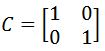# The Inverse of a 2 x 2 matrix

### The Inverse of a 2 x 2 matrix

In this lesson, we will learn how to find the inverse of a 2 x 2 matrix. You will learn that if two matrices are inverses of each other, then the product of the two matrices will result in an identity matrix. Next, you will learn how to find the inverse by using the formula below. You may find that the formula is hard to memorize. There is another way to find a 2 x 2 matrix without memorizing the formula, but it would require matrix row operations. You will see this method in the section "the inverse of 3 x 3 matrices with matrix row operations". Lastly, note that the inverse of a 2 x 2 identity matrix is just the identity matrix itself.

#### Lessons

Let the matrices $X$ and $Y$ be inverses. Then that means the following is true:
$XY=I$
where $I$ is the identity matrix. To be more precise, we can say that since $X$ and $Y$ are inverses, then $Y$ is the same as $X^{-1}$, and so we can say that
$XX^{-1}=I$

Let $X$be a matrix and you want to find the inverse (denote as $X^{-1}$ ). Then we use the following formula: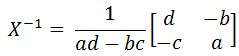• Introduction
The Inverse of a 2 x 2 matrix overview:
a)
Are the two matrices inverses?

b)
Finding the inverse of a 2 x 2 matrix

• 1.
Checking if the two matrices are inverses
Check that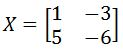and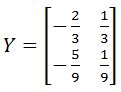are inverses.

• 2.
Check that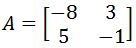and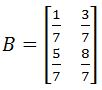are inverses.

• 3.
Check that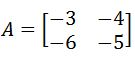and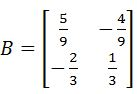are inverses.

• 4.
Finding the inverse of a matrix
Find the inverse of the matrix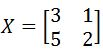• 5.
Find the inverse of the matrix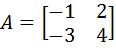• 6.
Find the inverse of the matrix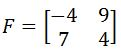• 7.
Find the inverse of the matrix